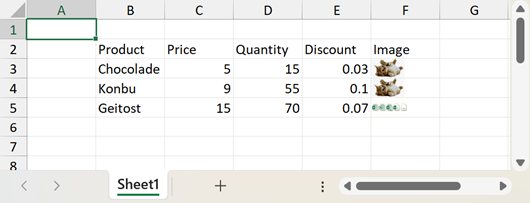The requested page is not available for the requested platform. You are viewing the content for .NET Framework 4.5.2+ platform.

## Mobile

19.2
19.1
18.2
18.1
17.2
.NET Framework 4.5.2+
.NET Framework 4.5.2+
.NET Standard 2.0+
.NET Core 3.0+

# WorksheetExtensions.Import(Worksheet, DataTable, Boolean, Int32, Int32) Method

Imports data from a data table.

You require a license to the DevExpress Office File API or DevExpress Universal Subscription to use this method in production code.

Assembly: DevExpress.Docs.v19.2.dll

## Declaration

``````public static void Import(
this Worksheet sheet,
DataTable source,
int firstRowIndex,
int firstColumnIndex
)``````
``````<ExtensionAttribute>
Public Shared Sub Import(
sheet As Worksheet,
source As DataTable,
firstRowIndex As Integer,
firstColumnIndex As Integer
)``````
``````public static void Import(
this Worksheet sheet,
DataTable source,
int firstRowIndex,
int firstColumnIndex
)``````
``````<ExtensionAttribute>
Public Shared Sub Import(
sheet As Worksheet,
source As DataTable,
firstRowIndex As Integer,
firstColumnIndex As Integer
)``````
``````public static void Import(
this Worksheet sheet,
DataTable source,
int firstRowIndex,
int firstColumnIndex
)``````
``````<ExtensionAttribute>
Public Shared Sub Import(
sheet As Worksheet,
source As DataTable,
firstRowIndex As Integer,
firstColumnIndex As Integer
)``````

## Parameters

Name Type Description
sheet Worksheet

A Worksheet that is the worksheet to which the data is imported.

source DataTable

A DataTable object that is the data source for import.

true, to insert column names to the row above the cells containing imported data; otherwise, false.

firstRowIndex Int32

An integer that is the row index of the start cell in which the imported data will be inserted.

firstColumnIndex Int32

An integer that is the column index of the start cell in which the imported data will be inserted.

## Parameters

Name Type Description
sheet Worksheet

A Worksheet that is the worksheet to which the data is imported.

source DataTable

A DataTable object that is the data source for import.

true, to insert column names to the row above the cells containing imported data; otherwise, false.

firstRowIndex Int32

An integer that is the row index of the start cell in which the imported data will be inserted.

firstColumnIndex Int32

An integer that is the column index of the start cell in which the imported data will be inserted.

## Parameters

Name Type Description
sheet Worksheet

A Worksheet that is the worksheet to which the data is imported.

source DataTable

A DataTable object that is the data source for import.

true, to insert column names to the row above the cells containing imported data; otherwise, false.

firstRowIndex Int32

An integer that is the row index of the start cell in which the imported data will be inserted.

firstColumnIndex Int32

An integer that is the column index of the start cell in which the imported data will be inserted.

## Remarks

The following code illustrates how to import data from a DataTable object to a worksheet. Note that the cell data types are set automatically, according to the data types of the source column. Cell formats are set automatically to the default value for the cell data type. However, you can easily change them, as described in the How to: Specify Number or Date Format for Cell Content topic.

``````Private Sub ImportDataTable()

Dim worksheet As Worksheet = spreadsheetControl1.Document.Worksheets(0)

' Create a "Products" DataTable object with four columns.
Dim sourceTable As New DataTable("Products")